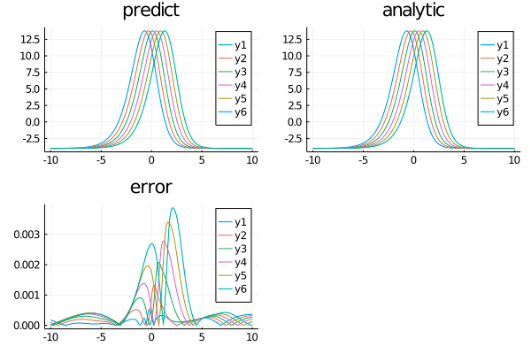# Kuramoto–Sivashinsky equation

Let's consider the Kuramoto–Sivashinsky equation, which contains a 4th-order derivative:

$$$∂_t u(x, t) + u(x, t) ∂_x u(x, t) + \alpha ∂^2_x u(x, t) + \beta ∂^3_x u(x, t) + \gamma ∂^4_x u(x, t) = 0 \, ,$$$

where \alpha = \gamma = 1 and \beta = 4. The exact solution is:

$$$u_e(x, t) = 11 + 15 \tanh \theta - 15 \tanh^2 \theta - 15 \tanh^3 \theta \, ,$$$

where \theta = 1 - x/2 and with initial and boundary conditions:

\begin{align*} u( x, 0) &= u_e( x, 0) \, ,\\ u( 10, t) &= u_e( 10, t) \, ,\\ u(-10, t) &= u_e(-10, t) \, ,\\ ∂_x u( 10, t) &= ∂_x u_e( 10, t) \, ,\\ ∂_x u(-10, t) &= ∂_x u_e(-10, t) \, . \end{align*}

We use physics-informed neural networks.

using NeuralPDE, Flux, ModelingToolkit, Optimization, GalacticOptimJL, DiffEqFlux
import ModelingToolkit: Interval, infimum, supremum

@parameters x, t
@variables u(..)
Dt = Differential(t)
Dx = Differential(x)
Dx2 = Differential(x)^2
Dx3 = Differential(x)^3
Dx4 = Differential(x)^4

α = 1
β = 4
γ = 1
eq = Dt(u(x,t)) + u(x,t)*Dx(u(x,t)) + α*Dx2(u(x,t)) + β*Dx3(u(x,t)) + γ*Dx4(u(x,t)) ~ 0

u_analytic(x,t;z = -x/2+t) = 11 + 15*tanh(z) -15*tanh(z)^2 - 15*tanh(z)^3
du(x,t;z = -x/2+t) = 15/2*(tanh(z) + 1)*(3*tanh(z) - 1)*sech(z)^2

bcs = [u(x,0) ~ u_analytic(x,0),
u(-10,t) ~ u_analytic(-10,t),
u(10,t) ~ u_analytic(10,t),
Dx(u(-10,t)) ~ du(-10,t),
Dx(u(10,t)) ~ du(10,t)]

# Space and time domains
domains = [x ∈ Interval(-10.0,10.0),
t ∈ Interval(0.0,1.0)]
# Discretization
dx = 0.4; dt = 0.2

# Neural network
chain = FastChain(FastDense(2,12,Flux.σ),FastDense(12,12,Flux.σ),FastDense(12,1))

discretization = PhysicsInformedNN(chain, GridTraining([dx,dt]))
@named pde_system = PDESystem(eq,bcs,domains,[x,t],[u(x, t)])
prob = discretize(pde_system,discretization)

callback = function (p,l)
println("Current loss is: \$l")
return false
end

opt = GalacticOptimJL.BFGS()
res = Optimization.solve(prob,opt; callback = callback, maxiters=2000)
phi = discretization.phi

And some analysis:

using Plots

xs,ts = [infimum(d.domain):dx:supremum(d.domain) for (d,dx) in zip(domains,[dx/10,dt])]

u_predict = [[first(phi([x,t],res.minimizer)) for x in xs] for t in ts]
u_real = [[u_analytic(x,t) for x in xs] for t in ts]
diff_u = [[abs(u_analytic(x,t) -first(phi([x,t],res.minimizer)))  for x in xs] for t in ts]

p1 =plot(xs,u_predict,title = "predict")
p2 =plot(xs,u_real,title = "analytic")
p3 =plot(xs,diff_u,title = "error")
plot(p1,p2,p3)